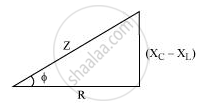PUC Karnataka Science Class 12Department of Pre-University Education, Karnataka
Share

# A Series Lcr Circuit is Connected to a Source Having Voltage V = Vm Sin ωT. Derive the Expression for the Instantaneous Current I and Its Phase Relationship to the Applied Voltage. - PUC Karnataka Science Class 12 - Physics

#### Question

A series LCR circuit is connected to a source having voltage v = vm sin ωt. Derive the expression for the instantaneous current I and its phase relationship to the applied voltage.

Obtain the condition for resonance to occur. Define ‘power factor’. State the conditions under which it is (i) maximum and (ii) minimum.

#### Solution

v = vm sin ωt

Let the current in the circuit be led the applied voltage by an angleΦ.

i= i_m sin(omegat +phi)

The Kirchhoff’s voltage law givesL ((di)/dt +Ri +q/C = v).

It is given that v = vm sin ωt (applied voltage)

L(d^2q)/(dt^2) +R(dq)/(dt) +q/C = v_m sinomegat    ...... (1)

On solving the equation, we obtain

q = q_m sin(omegat + theta)

(dp)/(dt) = q_momega cos(omegat +theta)

((d^2)q)/(dt^2)  = -q_momega^2 sin(omegat +theta)

On substituting these values in equation (1), we obtain

q_momega[R cos(omegat +theta)+ (X_c -X_L)sin(omegat +theta)] = v_msinomegat

X_c = 1/(omegaC)  X_c = omegaL

Z = sqrt(R^2 +(X_c - X_L)^2

q_momegaZ[R/Z cos(omegat+theta)+((X_c -X_L))/Z sin (omegat+theta)] = v_m sin omegat         ........... (2)

Let cos phi = R/2 and (X_c -X_L)/Z = sinphi

This gives

tan phi = (X_c - X_L)/R

On substituting this in equation (2), we obtain

q_momegaZcos (omegat +theta -phi) = v_msinomegat

On comparing the two sides, we obtain

V_m = q_momegaZ = i+mZ

i_m = q_momega

and (theta-phi) = -pi/2

I = (dp)/(dt ) =q_momega cos (omegat+theta)

=i_m cos(omegat+theta)

Or

i = i_m sin(omegat +theta)

Where,i_m = (v_m)/Z = (v_m)/(sqrt(R^2 +(X_c - X_L)^2)

And

phi = tan^-1((X_c -X_L)/R)

The condition for resonance to occur

i_m = v_m/sqrt(R^2 +(X_C - X_L)^2)

For resonance to occur, the value of im has to be the maximum.

The value of im will be the maximum when

X_c = X_L

1/(omega C) = omegaL

omega^2 = 1/(LC)

omega = 1/(sqrtLC)

2pif = 1/sqrt(LC)

f = 1/(02pisqrt(LC)

Power factor = cos ΦWhere,cosphi = R/Z = R/(sqrt(R^2 +(X_c- X_L)^2)

(i) Conditions for maximum power factor (i.e., cos Φ = 1)

• XC = XL

Or

• R = 0

(ii) Conditions for minimum power factor

• When the circuit is purely inductive

• When the circuit is purely capacitive

Is there an error in this question or solution?

#### Video TutorialsVIEW ALL 

Solution A Series Lcr Circuit is Connected to a Source Having Voltage V = Vm Sin ωT. Derive the Expression for the Instantaneous Current I and Its Phase Relationship to the Applied Voltage. Concept: Ac Voltage Applied to a Series Lcr Circuit.
S